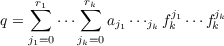#### Vol. 84, No. 1, 1979

 Recent Issues Vol. 325: 1  2 Vol. 324: 1  2 Vol. 323: 1  2 Vol. 322: 1  2 Vol. 321: 1  2 Vol. 320: 1  2 Vol. 319: 1  2 Vol. 318: 1  2Online Archive Volume: Issue:The Journal Subscriptions Editorial Board Officers Contacts Submission Guidelines Submission Form Policies for Authors ISSN: 1945-5844 (e-only) ISSN: 0030-8730 (print) Special Issues Author Index To Appear Other MSP Journals
Uniform and Lp approximation for generalized integral polynomials

### Le Baron O. Ferguson

Vol. 84 (1979), No. 1, 53–62
##### Abstract

Let X be a compact Hausdorff space and denote the space of all real valued continuous functions on X by C(X,R). With pointwise operations this space becomes a linear space which we norm by defining f= supxX|f(x)| whenever f C(X,R). A subset of C(X,R) is said to be point separating if for any two distinct points x and y in X there is an f in with f(x)f(y). Let Z[F] denote the ring of all polynomials in elements of which have integral coefficients. Thus an element q of Z[] is an element of C(X,R) with the special formwhere the a’s are integers, the f’s belong to and the r’s are nonnegative integers. Such q are our integral polynomials. If X is a subset of n-dimensional Euclidean space and is taken to be the set of n coordinate projections, then the elements of Z[] are polynomials in the usual sense.

Primary: 41A10
Secondary: 41A29
##### Milestones